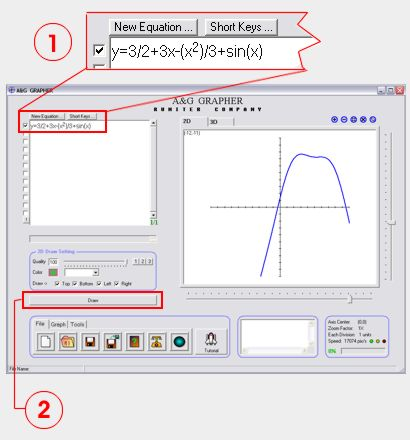A&G Grapher 5.80

 Product Overview How to use Screen shots What's new

 Get itIn order to plot the graph of an equation follow these simple steps:

(1) Type the equation in the equation box

As soon as you start typing a new equation, a check mark will automatically appear in the corresponding selection box. If you want to prevent an equation from being graphed, then simply uncheck the selection box.

For multiplication, you can omit the multiplication sign (*). For example instead of 2*x you can simply type 2x. Other examples include 2(x), 2(x+y) and 2xy.

If you want to raise an expression to a power of some number or expression (e.g. x2), use the ^ key. The equation editor will automatically shift the power part of the expression up. Press the Enter key to return to the base line.

When typing functions such as sin() or cos(), you only need to type the first letter and the editor will automatically fill-in the rest. The following table summarized the functions that are supported:

 Functions Short keys sin s cos c tan t asin (arcsine) as acos (arccosine) ac atan (arctangent) at abs (absolute value) ab log l e (constant e) e

(2) Click on Draw button

You can also press the shot-keys Ctrl+Enter to draw the equations. Other short-keys include Ctrl+'+' to zoom-in or Ctrl+'-' to zoom-out or Ctrl+'X' to reset the axis center.